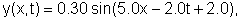# 1-5 Speed of a sinusoidal traveling waveA wave traveling along the x-axis is described by the equationwhere x and y are expressed in meters and t is in seconds. Find

1. the amplitude,

2. the wavelength,

3. the period,

4. the speed of the wave,

5. the direction of motion of the wave, and

6. the phase constant.

Example 2